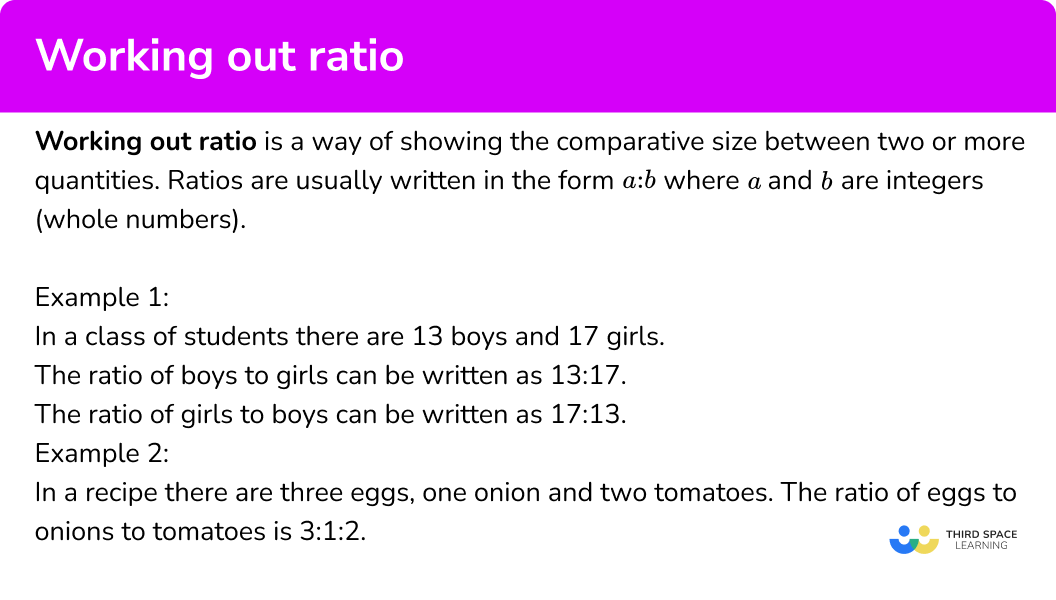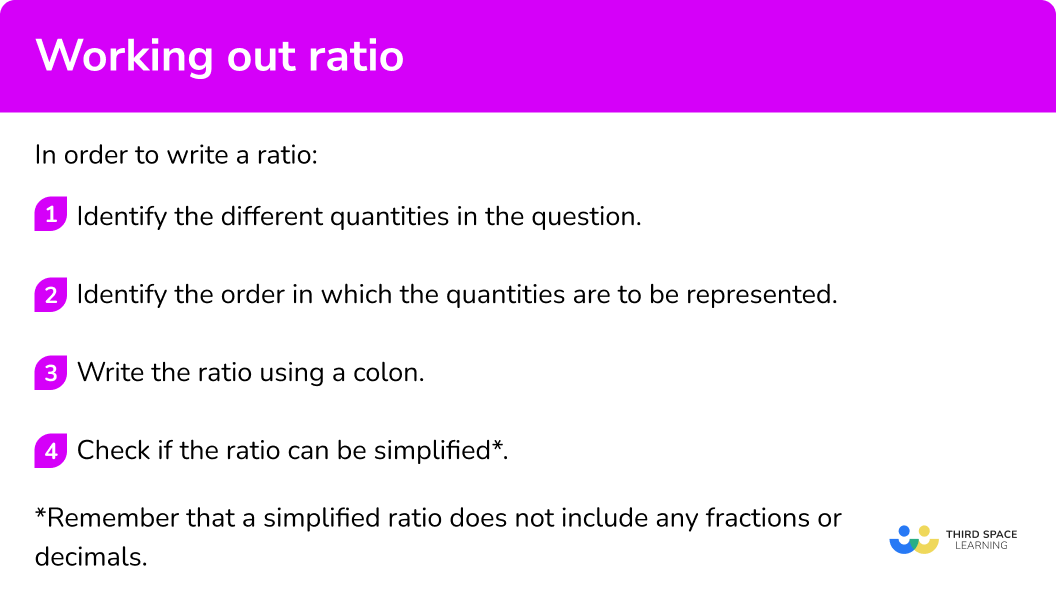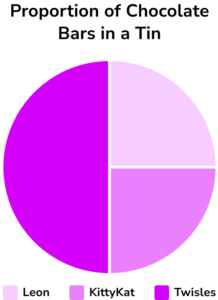# How To Work Out Ratio

Here we will learn about how to work out ratio, including how to write and simplify ratios.

There are also working out ratio worksheets based on Edexcel, AQA and OCR exam questions, along with further guidance on where to go next if you’re still stuck.

## What is working out ratio?

Working out ratio is a way of showing the comparative size between two or more quantities. Ratios are usually written in the form a:b where a and b are integers (whole numbers).

Example \bf{1}

In a class of students there are 13 boys and 17 girls.

The ratio of boys to girls can be written as 13:17.

The ratio of girls to boys can be written as 17:13.

Example \bf{2}

In a recipe there are three eggs, one onion and two tomatoes. The ratio of eggs to onions to tomatoes is 3:1:2.

Note: You can also write a ratio as a fraction in the form \frac{a}{b} (where a and b are integers) but this is less commonly used in the GCSE syllabus.

The ratio of boys to girls from example 1 would be  \frac{13}{17}.

The ratio of girls to boys from example 1 would be \frac{17}{13}.

The subject mentioned first is the numerator of the fraction and the subject mentioned second is the denominator.

Example \bf{3} : Here are some coloured squares.

Rearranging them into coloured bars, we can easily see the number of purple squares in comparison to the number of pink squares.

There are 7 purple squares and 5 pink squares. There are 12 squares in total.

The ratio of purple to pink squares is 7:5.

The ratio of pink to purple squares is 5:7.

The ratio of purple squares to the total number of squares is 7:12.

The ratio of pink squares to the total number of squares is 5:12.

Note: After you have written a ratio from a word problem, like in the examples above, you must then check if the ratio simplifies. When a ratio is fully simplified the parts of the ratio are all integers (whole numbers) with no common factors. This means a ratio must not include any decimals or fractions.

Step-by-step guide: Simplifying ratios

### What is working out ratio?## How to work out ratio

In order to work out ratio:

1. Identify the different quantities in the question.
2. Identify the order in which the quantities are to be represented.
3. Write the ratio using a colon.
4. Check if the ratio can be simplified*.

*Remember that a simplified ratio does not include any fractions or decimals.

### Explain how to work out ratio## Related lessons on ratio

How to work out ratio is part of our series of lessons to support revision on ratio. You may find it helpful to start with the main ratio lesson for a summary of what to expect, or use the step by step guides below for further detail on individual topics. Other lessons in this series include:

## How to work out ratio examples

### Example 1: writing a simple ratio about an everyday life situation

Ms Holly is looking after 7 children. She only has 5 toys in her nursery. Write the ratio of children to toys.

1. Identify the different quantities in the question.

There are 7 children.

There are 5 toys.

2Identify the order in which the quantities are to be represented.

Children to toys.

3Write the ratio using a colon.

children : toys

7:5

4Check if the ratio can be simplified.

This ratio is already in its simplest form.

### Example 2: writing a three part ratio about a real life situation

On a farm there are 20 pigs, 8 cows and 124 chickens. Write the ratio of cows to pigs to chickens in simplest form.

There are 20 pigs.

There are 8 cows.

There are 124 chickens.

Cows to pigs to chickens.

cows : pigs : chickens

8:20:124

8:20:124 (divide through by 4 to find the simplified equivalent ratio).

2:5:31

### Example 3: writing a ratio from a picture

Use the diagram below to write the ratio of pink counters to the total number of counters in simplest form.

There are 16 pink counters.

There are 24 counters all together.

Pink counters to the total number of counters.

pink : total

16:24

16:24 (divide through by 8 to find the simplified equivalent ratio).

2:3

### Example 4: writing a ratio from a graph

The comparative bar chart shows the number of left handed and right handed people in a group.

Write the ratio of left handed people to the total number of people in its simplest form.

There are 32 left-handed people.

There are 32+48 = 80 people in total.

Left handed people to the total number of people.

left handed : total

32:80

32:80 (divide through by 16 to find the simplified equivalent ratio).

2:5

### Example 5: writing a ratio from a word problem involving fractions

In a fridge there are three different types of drinks in bottles: water, coke and lemonade. \frac{3}{8} of the drinks are coke. \frac{1}{2} of the drinks are water. Write the ratio of water to coke to lemonade.

\frac{3}{8} of the drinks are coke.

\frac{1}{2} or \frac{4}{8} of the drinks are water. (A good technique when working with fractions is to write them all with the same denominator.)

1-\frac{3}{8}-\frac{4}{8}=\frac{1}{8} of the drinks are lemonade.

\frac{4}{8}:\frac{3}{8}:\frac{1}{8}

\frac{4}{8}:\frac{3}{8}:\frac{1}{8} (multiply through by 8 to find the simplified equivalent ratio).

4:3:1

### Example 6: writing an algebraic ratio from a word problem

Amy is \textbf{a} years old. Imran is 12 years older than Amy. Write the ratio of Imran’s age to Amy’s age.

Amy is \textbf{a} years old.

Imran is \bf{a + 12} years old.

Imran’s age to Amy’s age.

Imran : Amy

a+12:a

This ratio is already in its simplest form.

### Common misconceptions

• Writing the parts of the ratio in the wrong order

When a ratio is written, the order is very important. Remember to read the word problem carefully to ensure you get it right.

For example, there are 12 dogs and 13 cats. What is the ratio of cats to dogs?

A common mistake would be to write 12:13 because this is the order the numbers are presented in the information. However the question asks for the ratio of ‘cats to dogs’ so the correct answer is 13:12 because there are 13 cats and 12 dogs.

### Practice working out ratio questions

1. In a ball pool there are 258 red balls, 300 yellow balls and 546 blue balls. Write the ratio of blue balls to yellow balls to red balls in simplest form.

91:50:43258:300:546273:150:12931:25:21The parts of the ratio should be in the order blue : yellow : red.

First write 546:300:258.

By dividing through by 6 this simplifies to 91:50:43.

The correct ratio must have its parts in the correct order and be simplified.

2. A café sells 21 cups of coffee and 14 cups of tea during one lunch break. Write the ratio of coffee to tea sales in its simplest form.

21:143:53:221:3521 cups of coffee, 14 cups of tea.

Ratio of coffee to tea is 21:14.

By dividing through by 7 this simplifies to 3:2.

The correct ratio must have it’s part in the correct order and be simplified.

3. Matio is investigating how many people have the Facebook application on their phone. He stands outside a shop and conducts a survey on people he sees using a smartphone. 75 people report that they have the Facebook application.

25 people report that they do not have the Facebook application. Write the ratio of people who don’t have the Facebook application on their phone to the total number of people surveyed.

1:33:13:41:4The parts of the ratio should be in the order ‘no Facebook to total’.

Calculate the total 75+25 = 100.

Write the ratio 25:100.

By dividing through by 25 this simplifies to 1:4.

4. The pie chart shows the variety of chocolate bars in a tin. Write the ratio of Leon to KittyKats to Twisles in simplest form.1:290:90:1801:1:2\frac{1}{4}:\frac{1}{4}:\frac{1}{2}Leon has 90^o of the pie chart, KittyKat has 90^o of the pie chart and Twisles has 180^o of the pie chart.

Ratio of Leon to KittyKat to Twisles is 90:90:180 .

By dividing through by 90 this simplifies to 1:1:2.

5. On a bookcase there are F number of fiction books and N number of non-fiction books. Write the ratio of fiction books to the total number of books.

F:NF:FNF:N-FF:F+NThere are F+N books in total.

Ratio of fiction books to total number of books is F:F+N .

6. At a school \frac{1}{3} of students study French. The rest of the students study German. Write the ratio of students who study German to students who study French in simplest form.

2:1\frac{1}{3}:\frac{2}{3}1:33:4\frac{1}{3} of the students study French. The rest of the students study German which as a fraction is \frac{2}{3}.

Ratio of students who study German to students who study French is \frac{2}{3}:\frac{1}{3} (be careful of the order here).

By multiplying through by 3 this simplifies to 2:1.

### How to work out ratio GCSE questions

1. Robbie earns £8.40 per hour and Joseph earns £9.20 per hour. Robbie works for 5 hours each day, and Joseph works for 4.5 hours each day.

What is the ratio of Robbie’s daily pay to Joseph’s daily pay? Write your answer in its simplest form.

(2 marks)

Robbie’s earning for one day = 8.40 \times 5 = 42 .

Joseph’s earning for one day = 9.2 \times 4.5 =41.4 .

42 and 41.4

(1)

\begin{aligned} &42:41.4 \\\\ &420:414 \\\\ &70:69 \end{aligned}

(1)

2. An ice cream van sells three flavours of ice cream; vanilla, strawberry and chocolate. The pie chart shows the proportion of each flavour that was sold one hot summer’s day.(a) Write the ratio of chocolate to strawberry to vanilla for the ice cream sales shown in this pie chart.

(b) If 42 ice creams were sold in total, how many of them were chocolate?

(3 marks)

(a)

Chocolate = \frac{1}{6}, Strawberry = \frac{1}{3}, Vanilla = \frac{1}{2} / Chocolate 60^{o}, Strawberry 120^{o}, Vanilla 180^o

(1)

1:2:3

(1)

(b)

42 \div 6 = 7

(1)

3. Sammy, Yip and Harleen go to a sweet shop. Sammy buys twice as many sweets as Yip. Harleen buys 5 more sweets than Sammy. If Yip buys \textbf{n} sweets, write the ratio of Yip’s sweets to the total number of sweets.

(3 Marks)

Yip = n, Sammy = 2n, Harleen = 2n + 5

(1)

Total = n + 2n + 2n + 5 = 5n + 5

(1)

n:5n+5

(1)

## Learning checklist

You have now learned how to:

• Use ratio notation, including reduction to simplest form
• Express a multiplicative relationship between 2 quantities as a ratio or a fraction

## Still stuck?

Prepare your KS4 students for maths GCSEs success with Third Space Learning. Weekly online one to one GCSE maths revision lessons delivered by expert maths tutors.

Find out more about our GCSE maths tuition programme.